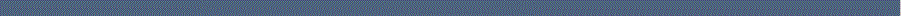Home Research interests Publications Conferences/Talks Supported projects since  2007 Supported projects  (1994 - 2006) Biographical Data

 The Kalman-Yakubovich-Popov Theorem in thermo-elastic problems
 The Kalman-Yakubovich-Popov Theorem for infinite-dimensional systems: Some new results a) Bounded control operator, C0-semigroups, Pritchard-Salamon systems Louis, J. Cl. and D. Wexler: The Hilbert space regulator problem and operator Riccati equation under stabilizability. Ann. Soc. Sci. Bruxelles, Ser. 1, 105, 1991, 137 -- 165. Van Keulen, B.: Equivalent conditions for the solvability of the nonstandard LQ-problem for Pritchard-Salamon systems. J. Control Optim., 33, 1995, 1326 -- 1356. Curtain, R. F.: The Kalman-Yakubovich-Popov Lemma for Pritchard-Salamon systems. System & Control Lett., 27, 1996, 67 -- 72. Curtain, R. F. and J. C. Oostveen: The Popov criterion for strongly stable distributed parameter systems. Int. J. Control, 74 (3), 2001, 265 -- 280. b) Parabolic systems, holomorphic semigroups, heat equation Pandolfi, L.: Dissipativity and Lur'e problem for parabolic boundary control systems. SIAM J. Control Optimiz., 36, 1998, 2061 -- 2081. Bucci, F.: Frequency domain stability of nonlinear feedback systems with unbounded input operator. Dynamics of Continuous, Discrete and Impulsive Systems, 7, 2000, 351 -- 368.c) First order hyperbolic equations Triggiani, R.: An optimal control problem with unbounded control operator and unbounded observation operator where the algebraic Riccati equation is satisfied as a Lyapunov equation. Appl. Math. Lett., 10 (2), 1997, 95 -- 102. d) Second order hyperbolic systems, Euler-Bernoulli equation, Kirchhoff equation, Schrödinger equation, string and membrane equation Lasiecka, I. and R. Triggiani: Algebraic Riccati equations arising in boundary / point control: A review of theoretical and numerical results. In: Perspective in control theory, Jacubczyk, B., Malanowski, K., Raspondek, W. eds., Birkhäuser, Boston, 1990. Part 1: Continuous case, 175 -- 210; Part 2: Approximation theory, 211 -- 235. Bucci, F.: The non-standard LQR problem for boundary control systems. Rend. Sem. Mat. Univ. Pol. Torino, 56 (4), 1998, 105 -- 114. Pandolfi, L.: The Kalman-Yakubovich-Popov Theorem for stabilizable hyperbolic boundary control systems. Integral Equations Operator Theory, 34, 1999, 478 -- 493. Barbu, V., Lasiecka, I. and R. Triggiani: Extended algebraic Riccati equations in the abstract hyperbolic case. Nonlinear. Anal. 40, 2000, 105 -- 129. e) Nonstandard Riccati equations arising in boundary control problems governed by damped wave and plate equations; Hammerstein integral equations with weekly singular kernels Flandoli, F.: Riccati equations arising in a boundary control problem with distributed parameters. SIAM Journal on Control and Optimization, 22, 1984, 76 -- 86. Lasiecka, I., D. Lukas and L. Pandolfi: Input dynamics and nonstandard Riccati equations with applications to boundary control of damped wave and plate equations. Journal of Optimization Theory and Applications, 84 (3), 1995, 549 -- 574. f) Discrete time distributed systems, approximation theory for boundary control systems Helton, J. W.: A spectral factorization approach to the distributed stable regulator problem; the algebraic Riccati equation. SIAM Journal on Control and Optimization, 14, 1976, 639 -- 661. Malinen, J.: Discrete time H^\infty algebraic Riccati equations. Techn. Report A 428, Institute of Mathematics, Helsinki University, Finland, 2000. Arov, D. Z., Kaashoek, M. A., and D. R. Pik: The Kalman-Yakubovich-Popov inequality and infinite dimensional discrete time dissipative systems. J. Operator Theory, 2005, to appear. g) Generalized (possibly unbounded) solutions of the KYP inequality Arov, D. Z. and O. J. Staffans: The infinite-dimensional continuous time Kalman-Yakubovich-Popov inequality. Operator Theory: Advances and Applications, 1, 2005, Birkhäuser Verlag Basel, 1 -- 28. h) Overview articles Pandolfi, L.: The Kalman-Popov-Yakubovich Theorem: an overview and new results for hyperbolic control systems. Nonlinear Analysis, Methods & Applications, 30 (2), 1997, 735 -- 745. Pandolfi, L.: Recent results on the Kalman-Popov-Yakubovich problem. Proc. Int. Conf. on Mathematics and its Applications, Yagyarta, 1999.